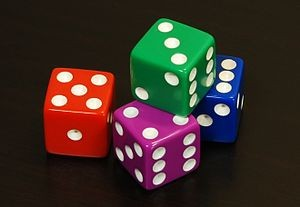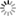Personal tools
You are here: Home Probability

Probability

- Overview

Probability theory is a mathematical framework for quantifying our uncertainty about the world. It allows us (and our software) to reason effectively in situations where being certain is impossible. Probability theory is at the foundation of many machine learning algorithms.

If The Weather Network informs you that the probability of precipitation is 80 per cent for the day, it might prompt you to carry an umbrella. We often use probability assessments informally in our daily lives to plan or make decisions. Formal probability theory is a fundamental tool used by researchers, health-care providers, insurance companies, stockbrokers and many others to make decisions in contexts of uncertainty.

Probability provides information about the likelihood that something will happen. Meteorologists, for instance, use weather patterns to predict the probability of rain. In epidemiology, probability theory is used to understand the relationship between exposures and the risk of health effects.

The beauty of probability theory is that it is applicable regardless of the interpretation of probability that we use (i.e., in terms of long-run frequency or degree of belief). Probability theory provides a solid framework to study random phenomena. It starts by assuming axioms of probability, and then building the entire theory using mathematical arguments.

- Randomness and Uncertainty

Randomness and uncertainty exist in our daily lives as well as in every discipline in science, engineering, and technology. Probability theory is a mathematical framework that allows us to describe and analyze random phenomena in the world around us. By random phenomena, we mean events or experiments whose outcomes we can't predict with certainty.

One way of thinking about "randomness" is that it's a way of expressing what we don't know. Perhaps if we knew more about the force I flipped the coin with, the initial orientation of the coin, the impact point between my finger and the coin, the turbulence in the air, the surface smoothness of the table the coin lands on, the material characteristics of the coin and the table, and so on, we would be able to definitively say whether the coin would come up heads or tails. However, in the absence of all that information, we cannot predict the outcome of the coin flip. When we say that something is random, we are saying that our knowledge about the outcome is limited, so we can't be certain what will happen.

- Probability and Statistics for Machine Learning

Probability is a measure of uncertainty. Probability applies to machine learning because in the real world, we need to make decisions with incomplete information. Hence, we need a mechanism to quantify uncertainty – which Probability provides us. Using probability, we can model elements of uncertainty such as risk in financial transactions and many other business processes. In contrast, in traditional programming, we work with deterministic problems i.e. the solution is not affected by uncertainty.

Basic concepts of probability and statistics are a must have for anyone interested in machine learning. Machine Learning is an interdisciplinary field that uses statistics, probability, algorithms to learn from data and provide insights which can be used to build intelligent applications.

Making predictions and searching for different structures in data is the most important part of data science. They are important because they have the ability to handle different analytical tasks. Therefore, statistics are a group of principles that are used to attain information about the data so as to make decisions. It unveils the secret hidden in the data.

Probability and Statistics are involved in different predictive algorithms that are there in Machine Learning. They help in deciding how much data is reliable, etc. Deep Learning is often called “Statistical Learning” and approached by many experts as statistical theory of the problem of the function estimation from a given collection of data.[Dice]

- Stochastic

The behavior and performance of many machine learning algorithms are referred to as stochastic. Stochastic refers to a variable process where the outcome involves some randomness and has some uncertainty. It is a mathematical term and is closely related to “randomness” and “probabilistic” and can be contrasted to the idea of “deterministic.”

The stochastic nature of machine learning algorithms is an important foundational concept in machine learning and is required to be understand in order to effectively interpret the behavior of many predictive models.

A variable is stochastic if the occurrence of events or outcomes involves randomness or uncertainty. Stochastic means that the model has some kind of randomness in it. A process is stochastic if it governs one or more stochastic variables. Games are stochastic because they include an element of randomness, such as shuffling or rolling of a dice in card games and board games.

In real life, many unpredictable external events can put us into unforeseen situations. Many games mirror this unpredictability by including a random element, such as the throwing of dice. We call these stochastic games.

- Stochastic Process Theory and Its Applications

In probability theory and related fields, a stochastic or random process is a mathematical object usually defined as a family of random variables.

Stochastic processes are widely used as mathematical models of systems and phenomena that appear to vary in a random manner. Examples include the growth of a bacterial population, an electrical current fluctuating due to thermal noise, or the movement of a gas molecule. Stochastic processes have applications in many disciplines such as biology, chemistry, ecology, neuroscience, physics, image processing, signal processing, control theory, information theory, computer science, cryptography and telecommunications. Furthermore, seemingly random changes in financial markets have motivated the extensive use of stochastic processes in finance.

Although stochastic process theory and its applications have made great progress in recent years, there are still a lot of new and challenging problems existing in the areas of theory, analysis, and application, which cover the fields of stochastic control, Markov chains, renewal process, actuarial science, and so on. These problems merit further study by using more advanced theories and tools.

Potential topics include but are not limited to the following:

• Stochastic models
• Random motions
• Queuing theory
• Renewal process theory and its application
• Stochastic differential equation and stochastic control
• Application of queuing theory in traffic engineering
• Application of Markov process in communication theory engineering
• Applications to risk theory, insurance, actuarial science and system risk engineering

[More to come ...]

Document Actions